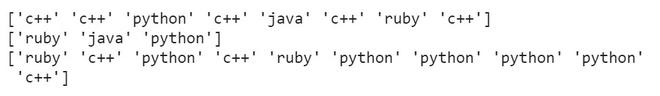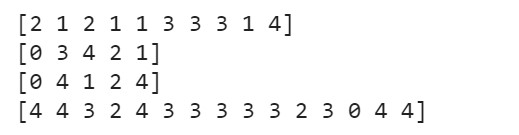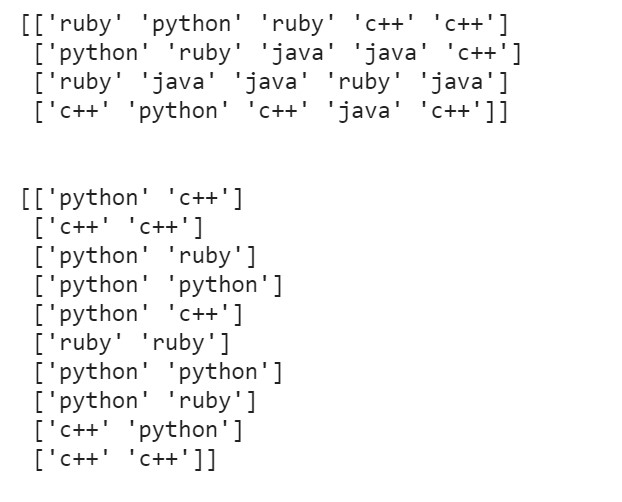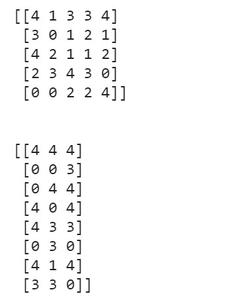# How to randomly select elements of an array with NumPy in Python ?

• Last Updated : 02 Dec, 2021

Prerequisites: Numpy

The random values are useful in data-related fields like machine learning, statistics and probability. The numpy.random.choice() function is used to get random elements from a NumPy array. It is a built-in function in the NumPy package of python.

Syntax: numpy.random.choice( a , size = None, replace = True, p = None)

Parameters:

• a: a one-dimensional array/list (random sample will be generated from its elements) or an integer (random samples will be generated in the range of this integer)
• size: int or tuple of ints (default is None where a single random value is returned). If the given shape is (m,n), then m x n  random samples are drawn.
• replace: (optional); the Boolean value that specifies whether the sample is drawn with or without replacement. When sample is larger than the population of the list, replace cannot be False.
• p: (optional); a 1-D array containing probabilities associated with each entry in a. If not given then sample assumes uniform distribution over all entries in a.

### Approach

• Import module
• Create a sample array
• Randomly choose values from the array created
• Print the array so generated.

Given below is the implementation for 1D and 2D array.

Generating 1-D list of random samples

Example 1:

## Python3

 `import` `numpy as np` `prog_langs ``=` `[``'python'``, ``'c++'``, ``'java'``, ``'ruby'``]` `# generating random samples``print``(np.random.choice(prog_langs, size``=``8``))` `# generating random samples without replacement``print``(np.random.choice(prog_langs, size``=``3``, replace``=``False``))` `# generating random samples with probabilities``print``(np.random.choice(prog_langs, size``=``10``,``                       ``replace``=``True``, p``=``[``0.3``, ``0.5``, ``0.0``, ``0.2``]))`

Output :Example 2:

## Python3

 `import` `numpy as np` `samples ``=` `5``# generating random samples``print``(np.random.choice(samples, size``=``10``))` `# generating random samples without replacement``print``(np.random.choice(samples, size``=``5``, replace``=``False``))` `# generating random samples with probabilities``print``(np.random.choice(samples, size``=``5``, replace``=``True``))` `# generating with probabilities``print``(np.random.choice(samples, size``=``15``,``                       ``replace``=``True``, p``=``[``0.2``, ``0.1``, ``0.1``, ``0.3``, ``0.3``]))`

Output:Generating a 2-D list of random samples

Example:

## Python3

 `import` `numpy as np` `prog_langs ``=` `[``'python'``, ``'c++'``, ``'java'``, ``'ruby'``]` `# generating random samples``print``(np.random.choice(prog_langs, size``=``(``4``, ``5``)))` `# generating random samples with probabilities``print``(``'\n'``)``print``(np.random.choice(prog_langs, size``=``(``10``, ``2``),``                       ``replace``=``True``, p``=``[``0.3``, ``0.5``, ``0.0``, ``0.2``]))`

Output:Example 2:

## Python3

 `import` `numpy as np` `samples ``=` `5` `# generating random samples``print``(np.random.choice(samples, size``=``(``5``, ``5``)))` `# generating with probabilities``print``(``'\n'``)``print``(np.random.choice(samples, size``=``(``8``, ``3``),``                       ``replace``=``True``,``                       ``p``=``[``0.2``, ``0.1``, ``0.1``, ``0.3``, ``0.3``]))`

Output:My Personal Notes arrow_drop_up